# How to Calculate and Solve for Discharge Current | Unconfined Aquifer | Water Budget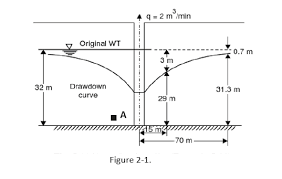The image above represents discharge current.

To compute for discharge current, five essential parameters are needed and these parameters are Hydraulic conductivity (k), Elevate from impermeable to surface (H), Depth of water in the well (hw), Radius of influence (R) and Radius of well (γw).

The formula for calculating discharge current:

Q = πk(H² – hw²) / In[R/γw]

Where:

Q = Discharge Current
k = Hydraulic Conductivity
H = Elevate from Impermeable to Surface
hw = Depth of Water in the Well

Let’s solve an example;
Find the discharge current when the hydraulic conductivity is 10,the elevate from impermeable to surface is 4, the depth of water in the well is 20, the radius of influence is 5 and the radius of well is 10.

This implies that;

k = Hydraulic Conductivity = 10
H = Elevate from Impermeable to Surface = 4
hw = Depth of Water in the Well = 20
R = Radius of Influence = 5
γw = Radius of Well = 10

Q = πk(H² – hw²) / In[R/γw]
Q = π(10)[(4)² – (20)²] / In[5/10]
Q = 31.415[16 – 400] / In[0.5]
Q = 31.415[-384] / -0.693
Q = -12063.7 / -0.69
Q = 17404.26

Therefore, the discharge current is 17404.26.

Nickzom Calculator – The Calculator Encyclopedia is capable of calculating the discharge current | Unconfined Aquifer.

To get the answer and workings of the discharge current | Unconfined Aquifer using the Nickzom Calculator – The Calculator Encyclopedia. First, you need to obtain the app.

You can get this app via any of these means:

To get access to the professional version via web, you need to register and subscribe for NGN 1,500 per annum to have utter access to all functionalities.
You can also try the demo version via https://www.nickzom.org/calculator

Apple (Paid) – https://itunes.apple.com/us/app/nickzom-calculator/id1331162702?mt=8
Once, you have obtained the calculator encyclopedia app, proceed to the Calculator Map, then click on Agricultural under Engineering.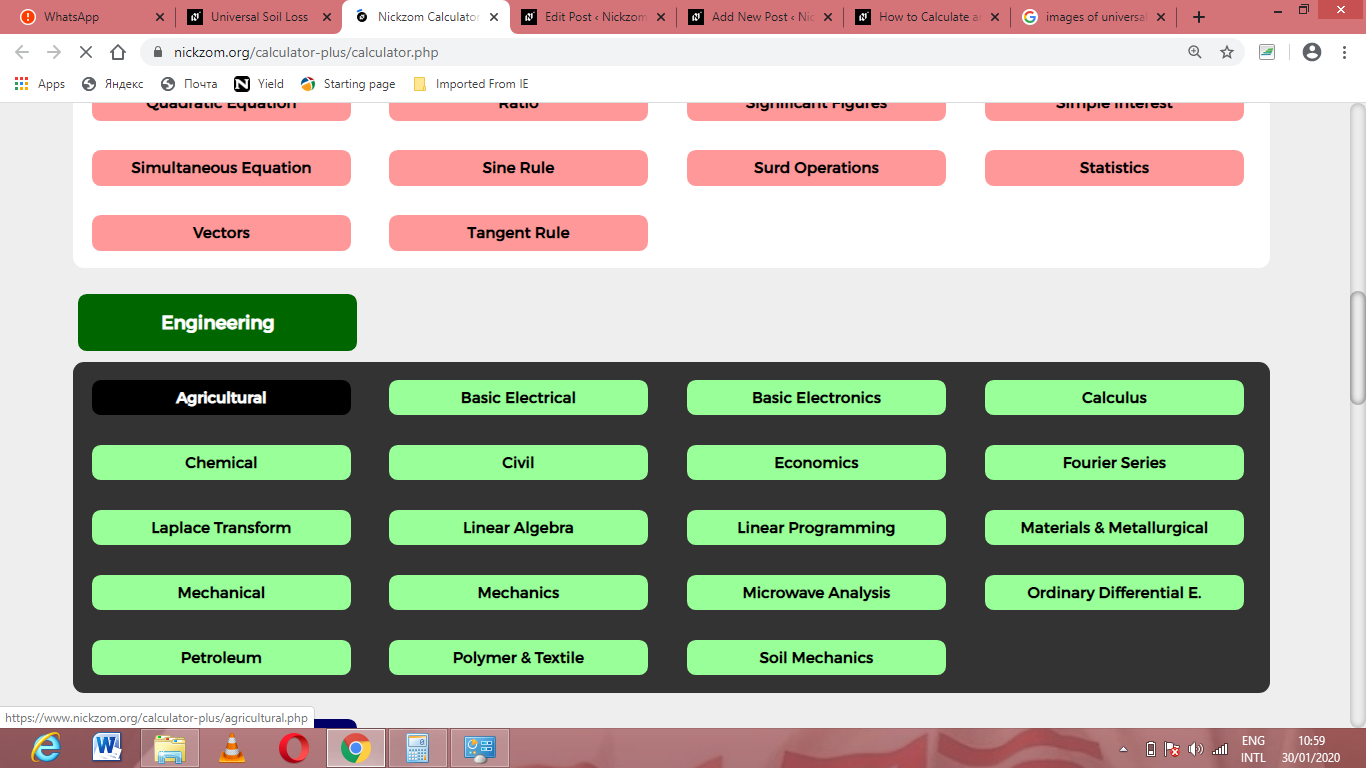Now, Click on Water Budget under Agricultural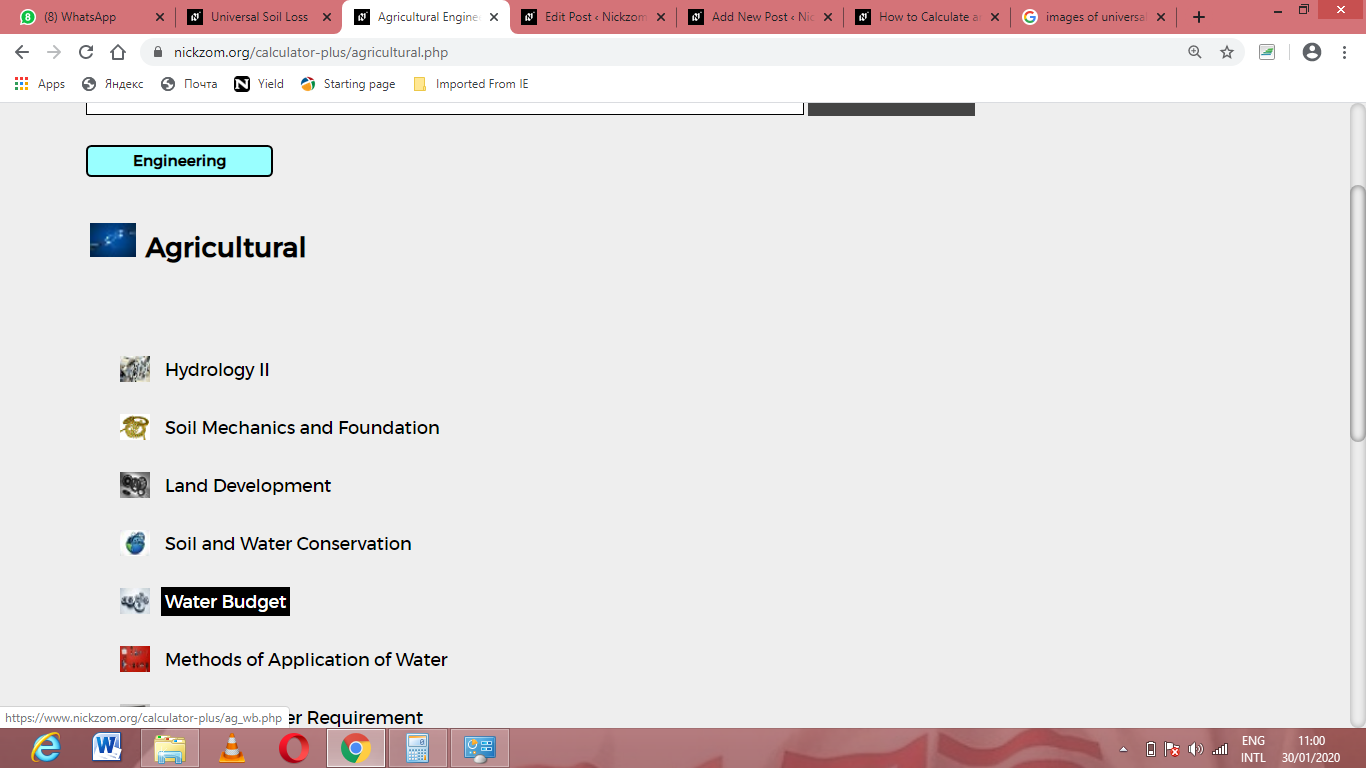Now, Click on Discharge Current | Unconfined Aquifer under Water Budget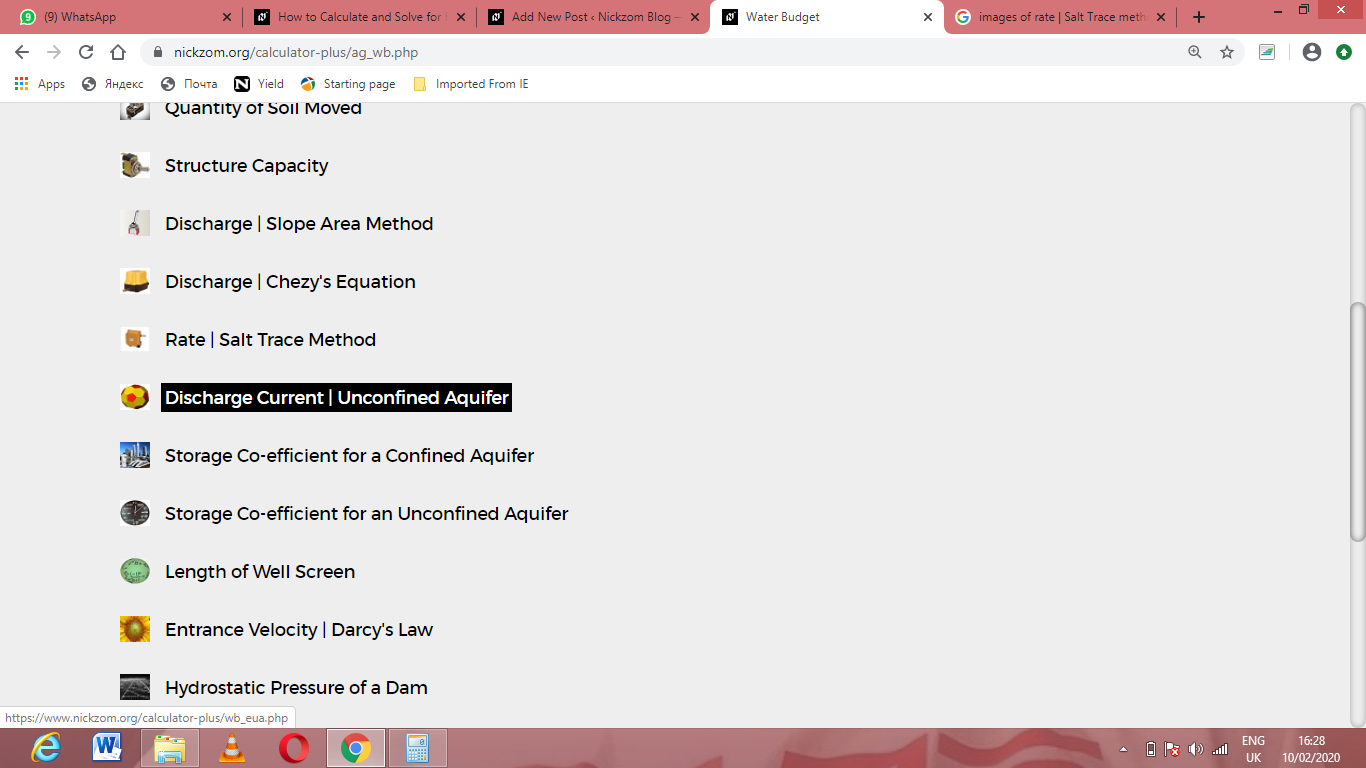The screenshot below displays the page or activity to enter your values, to get the answer for the discharge current | unconfined aquifer according to the respective parameters which are the Hydraulic conductivity (k), Elevate from impermeable to surface (H), Depth of water in the well (hw), Radius of influence (R) and Radius of well (γw).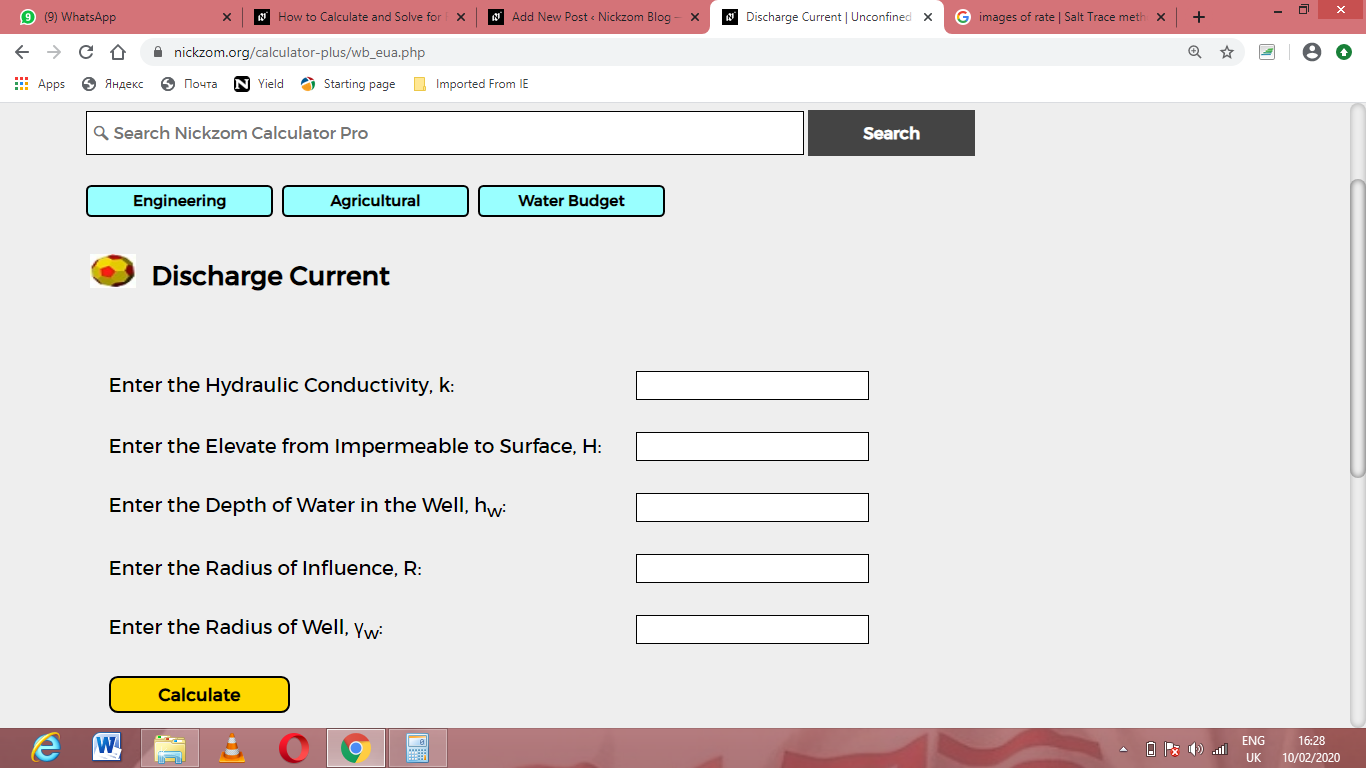Now, enter the values appropriately and accordingly for the parameters as required by the Hydraulic conductivity (k) is 10, Elevate from impermeable to surface (H) is 4, Depth of water in the well (hw) is 20, Radius of influence (R) is 5 and Radius of well (γw) is 10.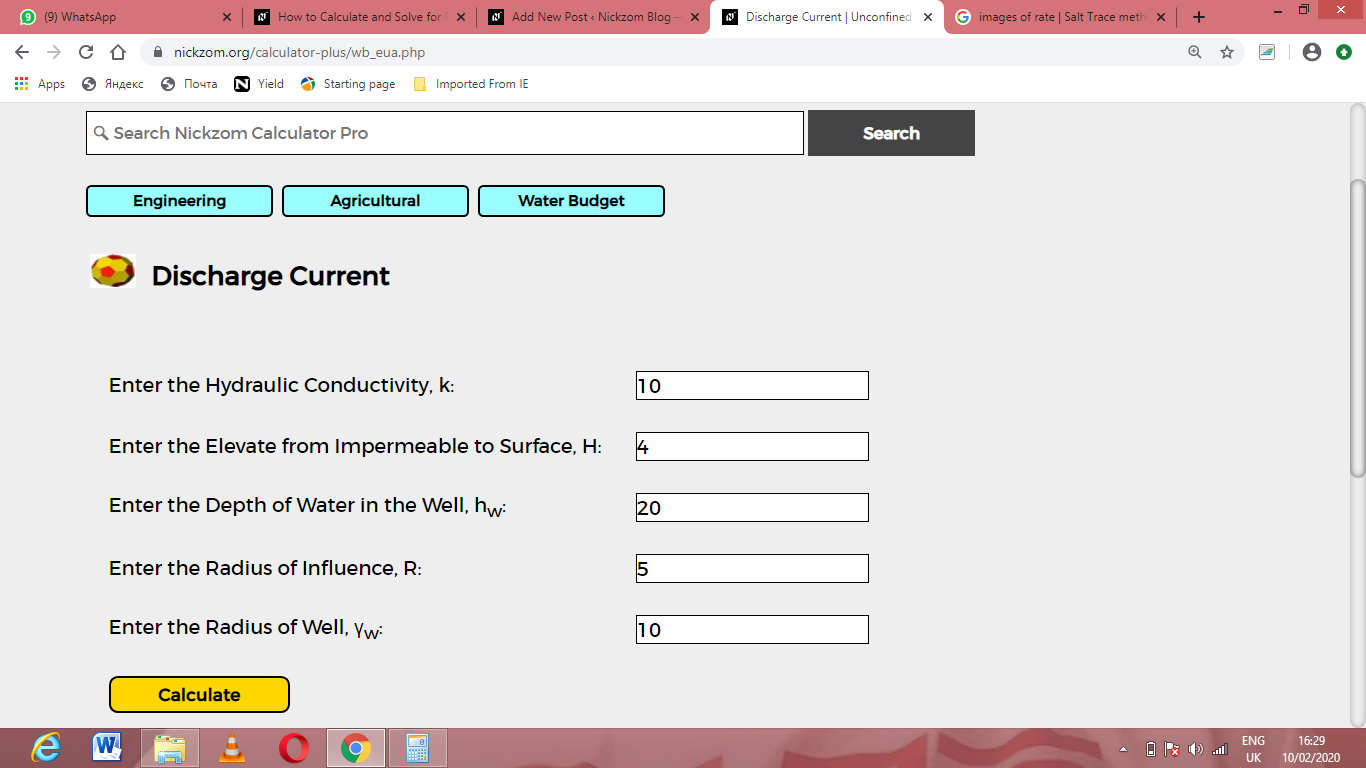Finally, Click on Calculate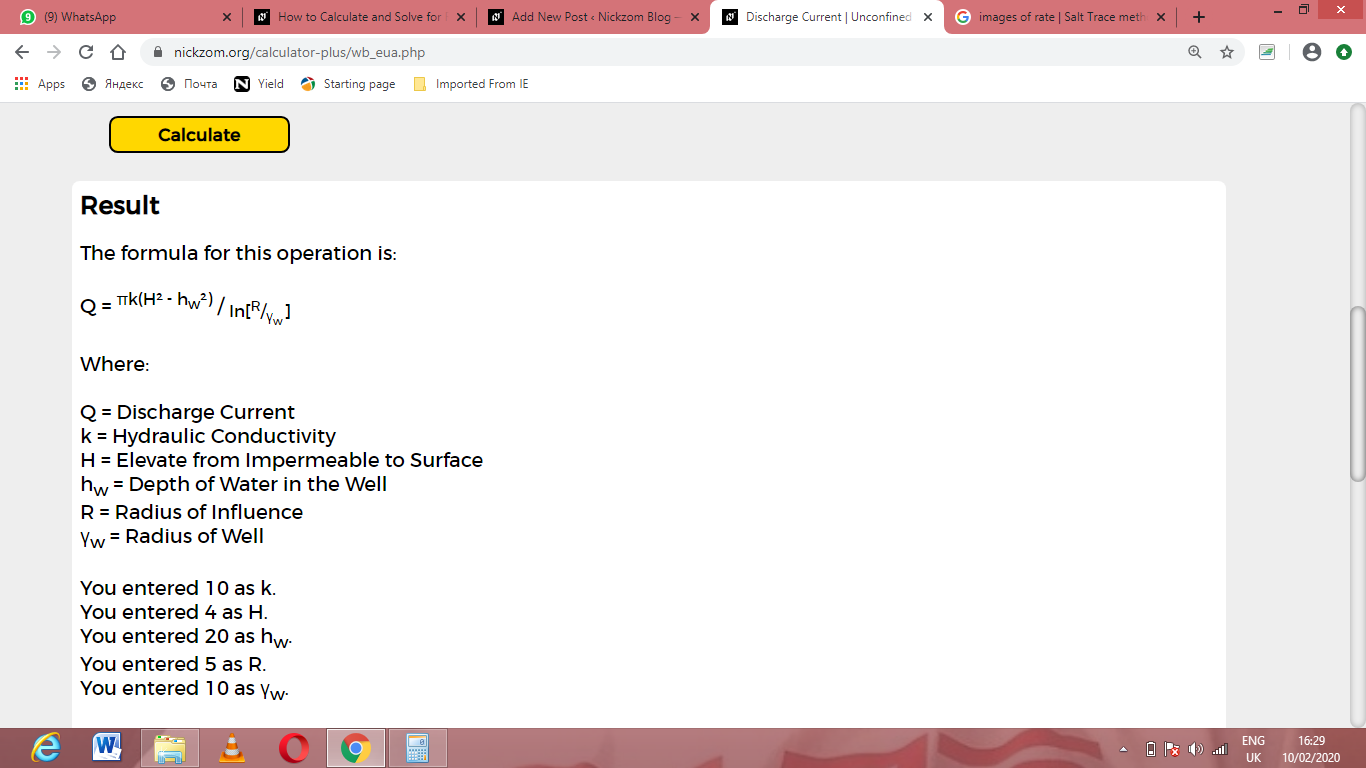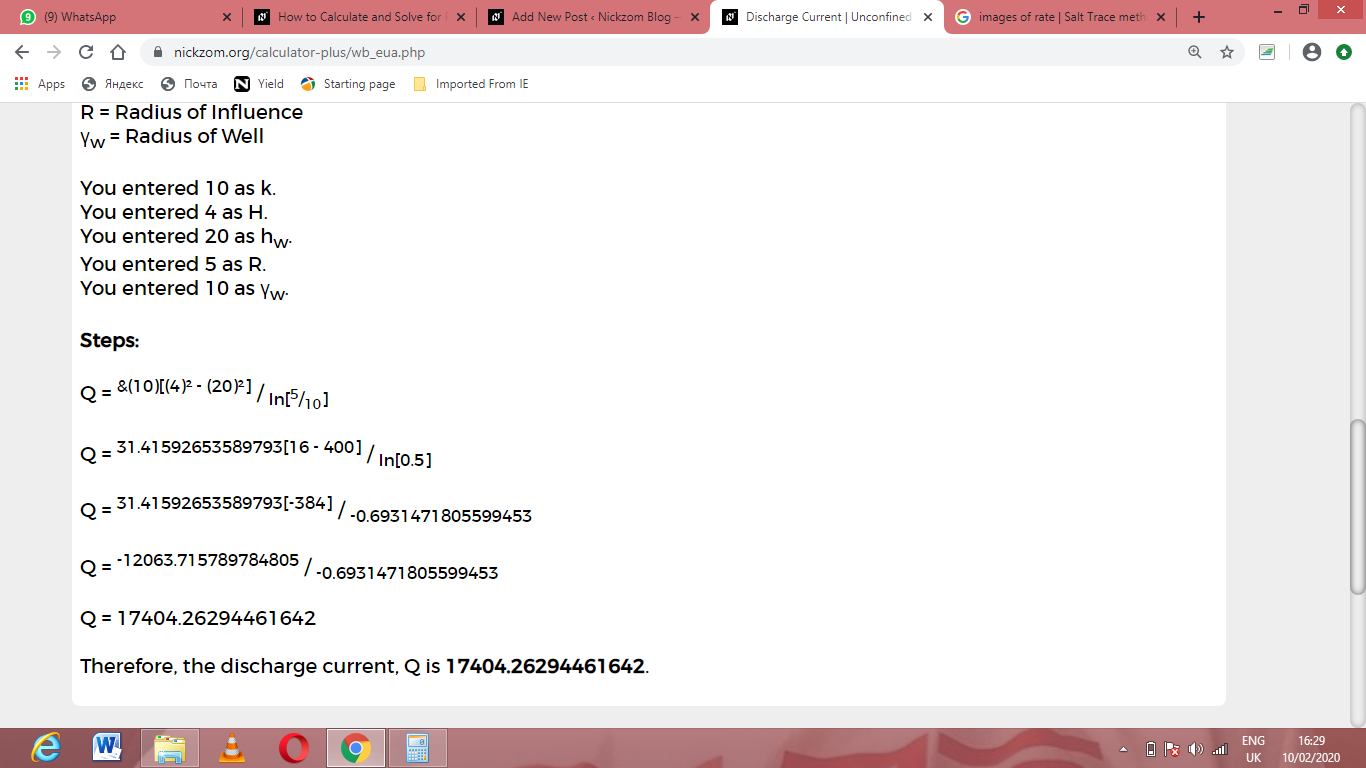As you can see from the screenshot above, Nickzom Calculator– The Calculator Encyclopedia solves for the discharge current | unconfined aquifer and presents the formula, workings and steps too.